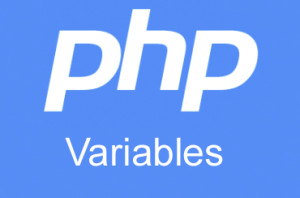# PHP Variables

by Lalita 24-Nov-15

0 1170

PHP Variables is very interesting, its just like a container. PHP Variables data transfer from one place to another place. PHP Variables start with \$ sign.Basic Example:

```<?php
\$a= 20;
echo \$a;
?>
```

Result: 20

There are two types of Variables :

1. Predefined Variable             2. User define Variables

1) Predefined Variables : These variables are available in PHP program. There is no need to declare and define these variable in the program. These are following type of Predefined Variable.

Predefined Variables = Super define variables.

\$_GET

\$_POST

\$_COOKIE

\$_SESSION

\$_FILES

\$_ENV

\$_REQUEST

\$_SERVER

2) User define Variable : This variable is declare and define by developers

For Example:

\$ontime=50;

\$ontime is a variable and 50 is a data and this data store in variable \$num. So we can use this variable for print the value of 50 at any where as per requirement.

Benefit of PHP Variable: PHP automatically converts the variable to correct data type, depending on value.

Example:

```<?php
\$a=30;
\$b=50;
\$c=\$a+\$b;
echo "First Number is \$a"."<br>";
echo "Second Number is \$b"."<br>";
echo "Result is \$c";
?>```
Result:
First Number is 30
Second Number is 50
Result is 80

Share:

## Comments

No Comments Yet.